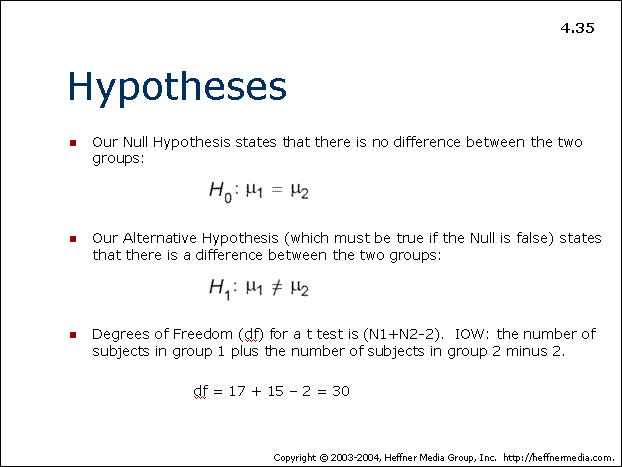# 35: Hypotheses -Null or Alternative## Hypotheses

• Our Null Hypothesis states that there is no difference between the two groups:

H0 : μ1 = &mu2

• Our Alternative Hypothesis (which must be true if the Null is false) states that there is a difference between the two groups:

H1 : μ1 ≠ μ2

• Degrees of Freedom (df) for a t test is (N1+N2-2). IOW: the number of subjects in group 1 plus the number of subjects in group 2 minus 2.

df = 17 + 15 – 2 = 30